## Vertical and FOIL Methods for Multiplying Two Binomials

### Learning Outcomes

• Use the FOIL method to multiply two binomials
• Use the vertical method to multiply two binomials

Remember that when you multiply a binomial by a binomial you get four terms. Sometimes you can combine like terms to get a trinomial, but sometimes there are no like terms to combine. Let’s look at the last example again and pay particular attention to how we got the four terms.

$\left(x+2\right)\left(x-y\right)$
${x}^{2}-\mathit{\text{xy}}+2x - 2y$

Where did the first term, ${x}^{2}$, come from?

It is the product of $x\text{ and }x$, the first terms in $\left(x+2\right)\text{and}\left(x-y\right)$.The next term, $-\mathit{\text{xy}}$, is the product of $x\text{ and }-y$, the two outer terms.The third term, $+2x$, is the product of $2\text{ and }x$, the two inner terms.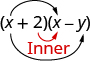And the last term, $-2y$, came from multiplying the two last terms.We abbreviate “First, Outer, Inner, Last” as FOIL. The letters stand for ‘First, Outer, Inner, Last’. The word FOIL is easy to remember and ensures we find all four products. We might say we use the FOIL method to multiply two binomials.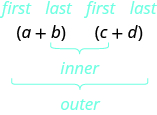Let’s look at $\left(x+3\right)\left(x+7\right)$ again. Now we will work through an example where we use the FOIL pattern to multiply two binomials.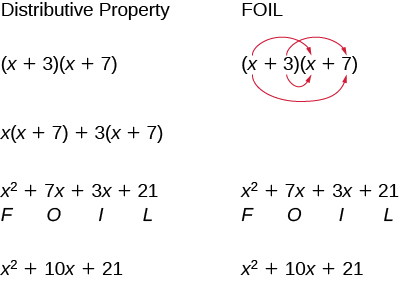### example

Multiply using the FOIL method: $\left(x+6\right)\left(x+9\right)$

Solution

 Step 1: Multiply the First terms.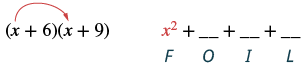Step 2: Multiply the Outer terms.Step 3: Multiply the Inner terms.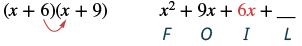Step 4: Multiply the Last terms.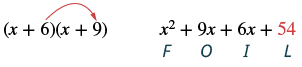Step 5: Combine like terms, when possible. $x^2+15x+54$

### try it

We summarize the steps of the FOIL method below. The FOIL method only applies to multiplying binomials, not other polynomials!

### Use the FOIL method for multiplying two binomials

1. Multiply the First terms.
2. Multiply the Outer terms.
3. Multiply the Inner terms.
4. Multiply the Last terms.
5. Combine like terms, when possible.### example

Multiply: $\left(y - 8\right)\left(y+6\right)$

### example

Multiply: $\left(2a+3\right)\left(3a - 1\right)$

### example

Multiply: $\left(5x-y\right)\left(2x - 7\right)$

### try it

For another example of using the FOIL method to multiply two binomials watch the next video.

## Multiplying Two Binomials Using the Vertical Method

The FOIL method is usually the quickest method for multiplying two binomials, but it works only for binomials. You can use the Distributive Property to find the product of any two polynomials. Another method that works for all polynomials is the Vertical Method. It is very much like the method you use to multiply whole numbers. Look carefully at this example of multiplying two-digit numbers.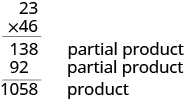You start by multiplying $23$ by $6$ to get $138$.

Then you multiply $23$ by $4$, lining up the partial product in the correct columns.

Last, you add the partial products.

Now we’ll apply this same method to multiply two binomials.

### example

Multiply using the vertical method: $\left(5x - 1\right)\left(2x - 7\right)$

### try it

We have now used three methods for multiplying binomials. Be sure to practice each method, and try to decide which one you prefer. The three methods are listed here to help you remember them.

### Multiplying Two Binomials

To multiply binomials, use the:

• Distributive Property
• FOIL Method
• Vertical Method

Remember, FOIL only works when multiplying two binomials.